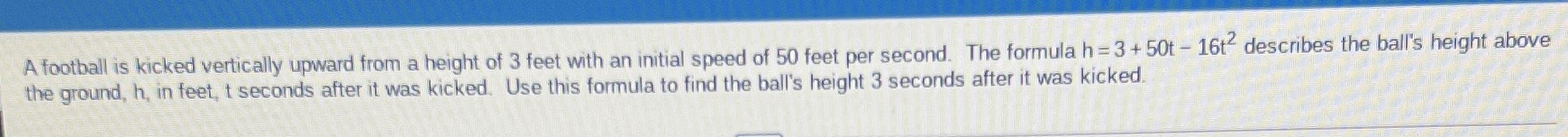### ¿Todavía tienes preguntas de matemáticas?

Pregunte a nuestros tutores expertos
Algebra
PreguntaA football is kicked vertically upward from a height of $$3$$ feet with an initial speed of $$50$$ feet per second. The formula $$h = 3 + 50 t - 16 t ^ { 2 }$$ describes the ball's height above the ground, $$h$$ , in feet, $$t$$ seconds after it was kicked. Use this formula to find the ball's height $$3$$ seconds after it was kicked.